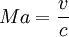# What is Mach 1

The Mach number (Ma) (named after the Austrian physicist and philosopher Ernst Mach) is a physical and dimensionless indicator of speed. It indicates the ratio of inertial forces to compression forces and is reduced to the ratio of the amount of a speed v (e.g. a body or a fluid) to the speed of sound c in the surrounding fluid. The following applies:.

Mach 1 is understood to be the speed of sound (which in exclusive Can assume different values ​​depending on the temperature). Correspondingly, “Mach 2” (twice the speed of sound), Mach 3, etc., cannot be converted into exact measures of speed either.

By means of the Mach number, however, flows can be divided into different areas, for example:

• M.a < 1="" subsonische="" strömung,="">
• 0,8 <>M.a < 1,2="" transsonische="" strömung,="">
• M.a > 1.2 supersonic flow.

From M.a > 5 one also speaks of hypersonic flow.

These areas require different approaches, since different physical phenomena occur in each case. For example, stand for M.a > 0.3 compressible effects in the currents (compressible flow), while such effects as a rule for M.a < 0,3="" keine="" rolle="" spielen.="">

### aviation

In aviation, the Mach number is used as a unit of measurement for the dimensionless indication of the true airspeed (TAS = True Airspeed), especially for fast-flying aircraft. Above an altitude of 26,000 feet (FL260 = FlightLevel = flight area, approx. 8 km), flights are usually flown according to the Mach number in international air traffic (regardless of the cruising speed of the respective aircraft). The flying mach number M.a is the ratio of airspeed to the speed of sound in the air; where the speed of sound depends exclusively on the temperature and not on the air pressure. The speed of sound at 25 ° C is about 346 m / s or 1245.6 km / h or c = 343 m / s for 20 ° C (room temperature), which corresponds to about 1,235 km / h.

At an altitude of 10,000 m at a temperature of -50 ° C, the speed of sound is 299.8 m / s = 1079.3 km / h. A passenger aircraft flying at a cruising speed of Mach 0.85 under these conditions has a speed of 254.83 m / s = 917.39 km / h.

### literature

• Götsch, Ernst - Aircraft technology, Motorbuchverlag, Stuttgart 2003, ISBN 3-613-02006-8
• Grossrubatscher, Michael - PilotsReference Guide, Munich 2007, ISBN 3-000-16686-6

Category: Unit of Measure